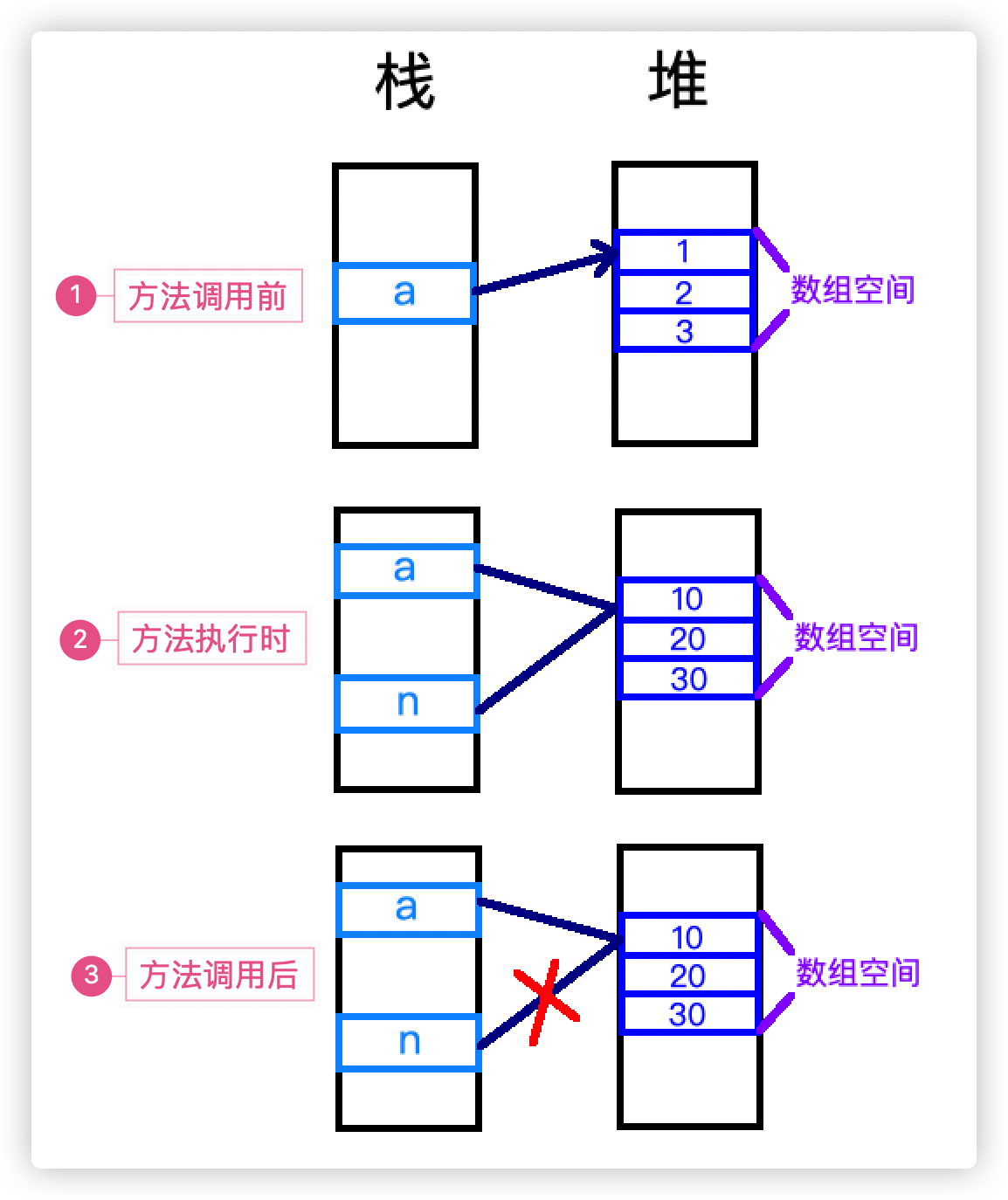# Java程序的方法设计

• 对于文章中出现的任何错误请大家批评指出，一定及时修改。
• 有任何想要讨论和学习的问题可联系我：zhuyc@vip.163.com。
• 发布文章的风格因专栏而异，均自成体系，不足之处请大家指正。

# Java程序的方法设计

## 一、方法的定义

### 1. 概念与作用

• 假如我们需要计算两个数的加和
public class Test{
public static void main(String[] args){
// 定义两个变量，so easy
int a = 10,b = 5;
int c = a + b;
System.out.println(c);// 15
}
}

• 如果我们需要多次反复执行同一个逻辑，那么就会产生很多相同的代码
public class Test{
public static void main(String[] args){
int a = 10,b = 5;
int c = 20,d = 10;
// 可以看到，虽然变量名称不同，但是计算的逻辑是相同的
// 如果某一段代码反复出现，我们可以考虑将他提取出来变成一个方法
int e = a + b;
System.out.println(e);// 15
int f = c + d;
System.out.println(f);// 30
int g = e + f;
System.out.println(g);// 45
}
}

• 定义方法后调用
public class Test{
public static void main(String[] args){
int a = 10,b = 5;
int c = 20,d = 10;
// 原有的代码逻辑将转变为方法的调用
plus(a,b);// 执行方法时输出：15
plus(c,d);// 执行方法时输出：30
plus(e,f);// 执行方法时输出：45
}
// 定义一个用于计算两个数加和的方法，计算后输出结果
public static void plus(int m,int n){
int result = m + n;
System.out.println(result);
}
}


• 从结构上来说，方法就是由多行代码所组成的集合
• 从使用的角度来看，定义方法的目的是抽取出通用的部分，可以减少重复代码的出现
• 从最终的效果来看，多行代码的执行转化为了方法的调用

### 2. 定义的格式

• 修饰符：对方法进行相关的限定，出现在返回值类型之前
• 权限修饰符：一般我们会将权限修饰符写在方法定义的最前面，它指明了这个方法都可以在什么地方被调用，最开始都声明为public即可
• 其他修饰符：可以修饰方法的关键词还有static，final等，会在其他文章中逐一介绍，修饰符的先后顺序没有严格要求
• 返回值类型：指明了方法执行后是否需要进行返回，以及相应的类型
• 方法名：指定方法的名称，方法被调用时使用，在同一类中同名方法将构成重载
• 参数列表：声明调用方法时需要传入的参数，可以为空，也可以多个
• 方法体：方法被调用时所执行的代码，是方法的核心部分，需要与方法的返回值类型呼应

### 3. 方法的签名

public class Test{
// 方法签名：main(String[] args)
public static void main(String[] args){
int a = 10,b = 5;
int c = plus(a,b);
}
// 方法签名：plus(int m,int n)
public static int plus(int m,int n){
return m + n;
}
}


### 4. 方法的注释

• 方法作用描述：描述方法的作用
• 方法参数描述：@param，解释每个参数代表的含义
• 返回类型描述：@return，解释返回值代表的含义

public class Test{
/**
* 计算两个数的加和
* @param a 第一个加数
* @param b 第二个加数
* @return 两个数的加和
*/
public int plus(int a,int b){
return a + b;
}
}


## 二、方法的设计

• What I want?

• What I need?

• How to do?

### 3. 参数列表的确定

• 声明的类型用于限制调用方法时传入参数的类型
• 声明的名称用于代表传递进来的参数

• 基本数据类型：对于基本数据类型，我们可以认为是值的传递，即：这是一个值拷贝之后，复制的过程，我们在方法中如果对参数的值进行修改，也不会改变原有的值。
public class Test{
public static void main(String[] args){
int a = 10;
test(a);// 进行方法的调用，方法中对值进行了修改
System.out.println(a);// 结果为10
}
public static void test(int n){
System.out.println(n);// 接收到值，结果为10
n = 100;// 修改n的值，不会影响传入的参数a的值
System.out.println(n);// 结果为100
}
}

• 引用类型：包括数组在内的引用类型，也就是除了基本数据类型以外的其他类型，在进行传递时发生的是引用传递，也就是说参数接收到的是一个引用，相当于多了一个变量指向了同一个位置，这样在方法中进行的修改直接会作用在对象实例上。
public class Test{
public static void main(String[] args){
int[] a = {1,2,3};
test(a);// 进行方法的调用，方法中对数组a进行了修改
for(int i = 0;i < a.length;i++){
System.out.println(n);// 结果为10,20,30
}
}
public static void test(int[] n){
for(int i = 0;i < n.length;i++){
System.out.println(n[i]);// 接收数组的引用，结果为:1,2,3
}
for(int i = 0;i < n.length;i++){
n[i] = n[i] * 10;// 修改数组的值，每个元素变为原来的10倍
}
// 对于修改对象的属性值同理，直接作用在对象本身
}
}• 可变参数：可变参数与数组类似，但是却有所不同，允许调用时以罗列的方式将参数传进来
• 可变参数又叫不定参数，从字面解释就是：有的时候我不确定参数到底有几个，但是又不想每次都构建一个数组，这个时候就可以使用不定参数
• 可变参数在一个方法的定义中只能出现一个
• 可变参数只能出现在参数列表的最后一个位置
• 不建议使用Object类型作为可变参数类型，将在方法重载时说明
• 声明格式：参数类型… 参数名称，如：int… nums
public class Test{
public static void main(String[] args){
int a = 1;
int b = 2;
int c = 3;
test(null);// 调用成功，此时参数为null
test();// 调用成功，此时参数个数为0
test(a);// 调用成功，传入1个参数
test(a,b);// 调用成功，传入2个参数
test(new int[]{a,b,c});// 调用成功，也可构建成数组后传入
}
public static void test(int... nums){
// 将nums当成数组一样使用即可，可以通过判断数组长度确定传入参数的个数
// 前提是传入的参数不为null，否则会出现空指针异常
if(nums == null){
System.out.println("传入的参数为null");
}else{
System.out.println("传入的参数个数为:" + nums.length);
}
}
}


### 4. 返回类型的确定

• void：代表方法执行后不需要指定返回值，也就是不需要使用return关键字，只需要完成方法的逻辑，输出某些信息，或者通过引用修改对象的某些属性。
• 其他类型
• 返回值类型只能指定一种，但可以是数组类型
• 如果声明了返回值类型，那么必须配合return关键字一同使用
• return在一般情况下只能出现在方法的最后一行，作为方法的结束
• 在选择结构中，也可能不会出现在最后一行的位置，可以根据需要提前结束某一个方法，但是必须保证选择结构对应的所有情况都有相应的返回值
• return后只能跟一个变量的名称或表达式，变量或表达式结果的类型必须和返回值类型相同
• 如果需要同时返回多个变量的值，可以使用数组
• 如果需要同时返回多种类型的变量，可以将返回值类型声明为：Object[]
public class Test{
public static void main(String[] args){
// 需要实现如下逻辑：计算两个数的加和，并将得到的结果变为10倍后输出
int a = 1,b = 2;
// 在进行方法调用后，我们必须想办法先得到两个数计算加和的结果，再继续下一步
int c = plus(a,b);
// 使用对应类型的变量（c）接收返回结果，然后继续下一步操作
int result = c * 10;
System.out.println(result);
}
public static int plus(int a,int b){
return a + b;
}
}


### 5. 方法内容的确定• 不要着急开始一个方法的编写
• 首先理清问题的解决步骤
• 如果可能，对每一个步骤进行细化，分析可能出现的情况，给出解决的办法
• 结合所学的语法知识，将每一个步骤翻译为相应的结构或代码
• 如果没有解决问题，重复以上步骤
• 经历几次之后你就可以完全在大脑中完成这几个步骤，顺畅的写出方法的内容

## 三、方法的调用

### 2. 方法的执行过程

public class Test{
public static void main(String[] args){
// 需要实现如下逻辑：计算两个数的加和，并将得到的结果变为10倍后输出
int a = 1,b = 2;// 代码执行步骤：1
// 代码执行步骤：2，进行方法的调用
int c = plus(a,b);// 代码执行步骤：4，进行返回值的赋值
int result = c * 10;// 代码执行步骤：5
System.out.println(result);// 代码执行步骤：6
}
public static int plus(int a,int b){
return a + b;// 代码执行步骤：3
}
}


### 3. 调用的注意事项

• static修饰符

static修饰符有很多作用，我们这里只讨论它用在方法上时，对方法的调用产生怎样的影响。由于main方法是程序的入口，那么它必须使用static声明，即：不需要实例化对象即可直接执行。那么由于main方法是static修饰的，那么它直接调用的方法必须也是由静态（static）修饰的。

• 接收返回值

## 四、方法的重载

### 1. 重载的概念

• 方法名称相同，参数列表不同

public class Test{
// 求两个整数和的方法：plus
public int plus(int a,int b){
return a + b;
}
// 参数列表相同，不构成重载，不能在类中同时存在
public int plus(int c,int d){
return c + d;
}
// 参数列表不同，构成重载
public double plus(double a,double b){
return a + b;
}
// 参数列表不同，构成重载，但是不定参数容易构成调用的歧义，不推荐
public int plus(int... a){
return 0;
}
// 参数列表相同，方法名称不同，不构成重载，可以在类中同时存在
public int Plus(int a,int b){
return a + b;
}
}

• 方法重载有什么用？

### 2. 重载方法的调用

public class Test{
public static void main(String[] args){
int a = 1,b = 2;
int c = plus(a,b);// 调用plus(int a,int b)
double m = 1.0,n = 2.0;
double d = plus(m,n);// 调用plus(double a,double b)
}
// 求两个整数和的方法：plus
public static int plus(int a,int b){
return a + b;
}
// 方法名相同，参数列表不同，构成重载
public static double plus(double a,double b){
return a + b;
}
}


public class Test{
public static void main(String[] args){
int a = 1,b = 2,c = 3;
int d = plus(a);// 编译失败，与plus(int... a)和plus(int a,int... b)都匹配
int e = plus(a,b);// 编译成功，调用plus(int a,int b)
int f = plus(a,b,c);// 编译失败，与plus(int... a)和plus(int a,int... b)都匹配
int g = plus(new int[]{a,b});// 编译成功，调用plus(int... a)
int h = plus(a,new int[]{b,c});// 编译成功，调用plus(int a,int... b)
}
// 求两个整数和的方法：plus
public static int plus(int a,int b){
return a + b;
}
// 方法名称相同，参数列表不同，构成重载，但是不定参数容易构成调用的歧义，不推荐
public int plus(int... a){
return 0;
}
// 方法名称相同，参数列表不同，构成重载，但是不定参数容易构成调用的歧义，不推荐
public int plus(int a,int... b){
return 0;
}
}10-244632
09-141万+
08-296739
11-281万+
10-113397
07-26692
07-084225
06-172372
06-121059
06-053017